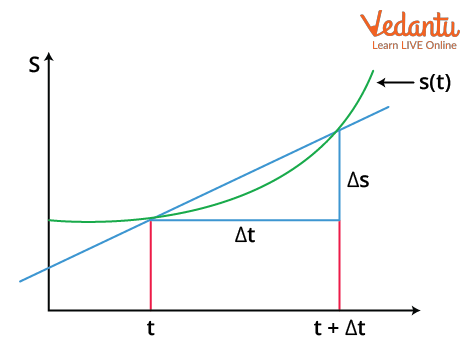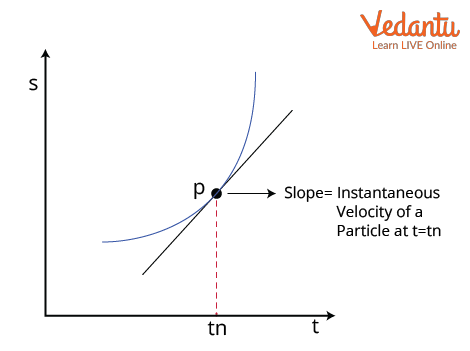Courses
Courses for Kids
Free study material
Free LIVE classes
More

# Instantaneous Velocity - Formula based Examples for JEELIVE
Join Vedantu’s FREE Mastercalss

## Instantaneous Velocity: Definition

The velocity of an object in motion at a certain point in time is termed instantaneous velocity simply put we define instantaneous velocity as a vector that is a measure of the speed at a particular time and not an interval of time. For an in-depth knowledge of instantaneous velocity, it is important to first understand the basic difference between velocity and speed. Velocity as we know is a vector quantity and is defined as the rate of change of displacement. However, speed on the other hand is a scalar quantity. Being a scalar quantity, speed only has magnitude and no direction.

We measure velocity in a fixed frame of reference. Therefore, speed and velocity are two different things. Additionally, velocity can be measured in two ways: average velocity and instantaneous velocity.

Average velocity is nothing but the ratio of displacement of an object between two points. As the name suggests we take an average of a given number of velocities to calculate the average velocity.

## Instantaneous Velocity Formula

Instantaneous velocity, also sometimes termed as simple velocity, is the measure of how fast an object or a body is moving at a particular instant along its path.

Instantaneous velocity is calculated as follows:

The rate of change of velocity w.r.t time is termed as average velocity and is denoted as: $\dfrac{ds}{dt}$

As velocity is to be calculated for the shortest instant of time thus time interval $\Delta t\rightarrow 0$

We finally calculate the instantaneous velocity as

$V_i=\displaystyle \lim_{\Delta t \to 0}{\dfrac{ds}{dt}}$

The SI unit of instantaneous velocity is

$\dfrac{meters}{seconds}$ or $\dfrac{m}{s}$

## Instantaneous Velocity Example

Some of the examples for which we can calculate instantaneous velocity are listed below

1. Fruits falling from the Branch of a Tree

Fruits falling from the trees reach the ground at different points and at different velocities. Here the instantaneous velocity of the fruit can be calculated at any point before it reaches the ground.

2. Speedometer

A speedometer or a speed meter is a perfect example to know instantaneous velocity. A speedometer is nothing but a device that is to measure the velocity of a vehicle at a particular second or time.

As we know, vehicles move at variable speeds at all times, therefore we can easily calculate the instantaneous velocity with the help of a speedometer.

3. A Person Jogging at Variable Speeds

A person will not jog at a constant velocity on a track. Thus, in this case, we can calculate the instantaneous velocity of the person by the formula mentioned above.

4. An Elevator

An elevator is another best example to define instantaneous velocity. An elevator reaches different floors at different times. As it does not move with a uniform velocity, thus, for a particular time, we can calculate the instantaneous velocity for an elevator using the above formula.

## Instantaneous Velocity Graph

The graph below shows the graph of position (s) versus time (t). To find the instantaneous velocity we assume t1 and t2 to be t and $t+\Delta t$ respectively.

Now we know that the instantaneous velocity is the limit of the average velocity as the time elapsed is zero. Thus, if we substitute the time values in the average velocity expression we can find the value of instantaneous velocity.

The expression for average velocity from the graph below will be given as

Putting t1 and t2, we get;

$V_i=\displaystyle \lim_{t \to 0}{\dfrac{s(t+\Delta t)-s(t)}{t+\Delta t-t}}$,

where $\Delta s$ and $\Delta t$ represents the change in position to the time elapsed as depicted in the graph below.Plot of instantaneous velocity

The graph is plotted for a moving body at different positions and times. The change in displacement is obviously due to change in velocity of the body. Hence, the curve s(t) is plotted accordingly. We take any two points on the curve corresponding to their time periods  t and $t+\Delta t$ and calculate the instantaneous velocity from the formula given above.

## Instantaneous Speed

The magnitude of instantaneous velocity at any given time is defined as the magnitude of instantaneous speed. The instantaneous speed is a scalar quantity and is always positive as compared to instantaneous velocity which can be positive or negative. Instantaneous speed is denoted as (v).

## Instantaneous Speed Formula:

The formula to calculate instantaneous speed is as below:

$V=\dfrac{ds}{dt}$

## Average Velocity Definition

Average velocity is defined as the displacement of an object or a body in time t. It is nothing but the average value of the known velocities. Average velocity is denoted as Vav. We can calculate the average velocity by dividing the total displacement traversed by a body or an object by the total time. The SI unit if average velocity is $\dfrac{meters}{seconds}$ or $\dfrac{m}{s}$.

The examples of average velocity are as listed below:

• A Person Travelling on a Bike.

A person riding a bike traverses through several different lengths of roads, therefore the displacement changes and so does the velocity. In this case to calculate the average velocity we need to consider the velocity both at starting and final points.

• An Athlete Training for a Marathon

An athlete or a person running on a track will change his speeds several times during the run. The average velocity here can be directly calculated as total displacement to the total time elapsed by the runner.

## Average Velocity Equation:

The average velocity is calculated using the formula below:

$V_{av}=\dfrac{X_f-X_i}{T_f-T_i}$

where,

Xf = final distance, Xi = initial distance

Tf = final time and Ti  = initial time

## Instantaneous Velocity vs Average Velocity

Now that we have looked at instantaneous velocity and average velocity separately, let us look at some basic differences between the two.

 S.No Instantaneous Velocity Average Velocity 1. Instantaneous velocity is the velocity of an object or body at a particular time. Average velocity is the total displacement of a body with a defined time interval. 2. Instantaneous velocity is a vector quantity. Average velocity is also a vector quantity 3. Instantaneous velocity is calculated as the ratio of average velocity to the minimum time period. Average velocity is the ratio of the rate of displacement to the time elapsed. 4. Some examples of instantaneous velocity are speedometers, elevators, fruit falling from a tree and so on. Some examples of average velocity are a person riding a bike, a Ferris wheel and so on.

## Instantaneous Velocity Problems

Problem 1: The movement of the bulldozer is given in the form of function s = t2 + 5t + 25. Measure its Instantaneous Velocity at time t = 3s.

Solution:

Here the given function of motion is s = t+ 5t + 25.

Now we have to differentiate the above function with time, then we get

$V_i=\dfrac{ds}{dt}=\dfrac{d(t^2+5t+25)}{dt}$

Vi = 2t + 5 =Vi(t)

For time t = 3s, the Instantaneous Velocity is measured as,

Vi (3) = 2(3) + 5

Vi (3) = 11 m/s

Thus, for the given function, the Instantaneous Velocity is 11 m/s.

Problem 2: The equation of motion gives the movement of a vehicle as Y = M t2 + n, where n = 12 m and M = 6 m. Calculate the instantaneous velocity at t = 4 seconds?

Solution:

The equation given is:

Y(t) = M t2 + n

$V_i(t)=\dfrac{ds}{dt}=2Mt$

Vi(t) = 2 M t

For, M = 6m   and t = 4s,

Vi(2) = 2 x 6 x 4 = 48 m/s

Vi(t) = 48 m/s

Therefore, Vi (instantaneous velocity)  is 48 m/s.

Problem 3: An object falls under the influence of gravity. Its equation of motion is given by S (t) = 6.3 t2. Calculate the instantaneous velocity of the body at the sixth second after the object is released under the influence of gravity.

Solution

The equation of motion is given as S (t) = 6.3 t2

For Instantaneous velocity at t = 6s is given by:

$V_i={\dfrac{ds}{dt}}_{t\rightarrow6}$

$V_i={\dfrac{6.3t^2}{dt}}_{t\rightarrow6}$

$V_i=6.3 x 2 x 6 = 75.6\,m/s$

## Conclusion

The article gives a complete walkthrough of the different velocities of an object and a thorough differentiation between all of them. It also explains the different concepts of average speed, average velocity, instantaneous velocity and instantaneous speed. It can be seen from above that all the quantities are somewhat interlinked with one another.

Last updated date: 23rd Sep 2023
Total views: 137.7k
Views today: 1.37k

## FAQs on Instantaneous Velocity - Formula based Examples for JEE

1. Is the instantaneous velocity a tangent to the curved path?

Yes, when calculating instantaneous velocity from a displacement versus time graph we draw a small line passing through a point at the tangent of a curved path. The slope of this line segment is the measure of the instantaneous velocity at a particular instant of time. Refer to the graph below for a clear understanding of the calculation of instantaneous velocity. Here tn represents the particular instant of time where the velocity is to be calculated.  The graph indicates the method to measure the instantaneous velocity when a displacement versus time graph is given.Calculation of Slope of Distance vs Time

2. Can Instantaneous velocity be negative? How is it different from instantaneous speed?

Yes, instantaneous velocity can be both positive and negative. Mathematically speaking a positive velocity indicates the direction of a body or an object is far away from the origin, whereas a negative direction indicates that the direction is towards the origin. Additionally, instantaneous speed is the magnitude of the instantaneous velocity which can also be denoted as |Vi|. As instantaneous speed is the magnitude therefore it is always positive. However, instantaneous velocity can be both positive and negative.